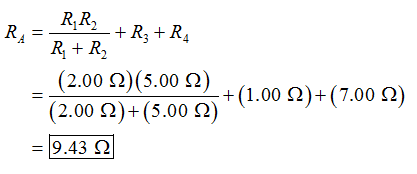Question
343 views

Consider the network of four resistors shown in the diagram, where R1= 2.00 Ω , R2= 5.00 Ω , R3= 1.00 Ω , and R4= 7.00 Ω . The resistors are connected to a constant voltage of magnitude V.

Part A: Find the equivalent resistance RA of the resistor network.

Part B: Two resistors of resistance R5= 3.00 Ω and R6= 3.00 Ω are added to the network, and an additional resistor of resistance R7= 3.00 Ω is connected by a switch, as shown in the diagram..(Figure 2) Find the equivalent resistance RB of the new resistor network when the switch is open.

Part C: Find the equivalent resistance RC of the resistor network described in Part B when the switch is closed.

check_circle

Step 1

(A)

From the figure (1), the resistors of the resistance R1 and R2 are connected in parallel combination with the resistors of the resistance R3 and R4 in series. The equivalent resistance (RA) of all these resistors can be calculated as,Step 2

(B)

From the figure (2), when the switch is open, no current flows through the resistor of the resistance R7. Thus, it will be neglected.

The resistors of the resistance R1 and R6 are connected in series, and these are connected with resistor of the resistance R2 in parallel combination. Then, these are connected with the resistors of the resistance R3, R4 and R5 in series. The equivalent resistance (RB) of a...

### Want to see the full answer?

See Solution

#### Want to see this answer and more?

Solutions are written by subject experts who are available 24/7. Questions are typically answered within 1 hour.*

See Solution
*Response times may vary by subject and question.
Tagged in